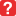Adding Dynamic Component Function to calculate lineal feetAdding Dynamic Component Function to calculate lineal feet

I am using Sketchup 8 to do bills of quantities, material measurement and calculate pay for tradesmen based on measured work. I got help from TIG (a forum moderator)in creating a Ruby code which added a volume function "volume()" to SU's DC function set. This was very useful in creating dynamic component attributes for entities such as walls, foundations and slabs in estimating the quantity of concrete to order from suppliers. This also helped with determining how varying the thickness of walls or adding an extra room would affect material quantities and overall cost. The FACEAREA dc function was also useful in calculating square footage for tiling, painting etc. However, when I need to calculate running feet, there is great difficulty. For example, if I need to calculate the total running feet of moulding strips along walls, then I could not use the Lenx or Leny functions as these only give the rectangular dimension of the entity box. What I need is the running feet of walls by floor plan. I need a function that would calculate the total lineal feet in the following ways:

1 Net Lineal Feet one side - Runninng feet at the base of the wall less any openings for doors etc for the inside or outside of a wall.
2 Net Lineal Feet one side - Runninng feet at the base of the wall less any openings for doors etc for both sides of the wall.
3 Gross Lineal Feet one side- Running feet at the base from intersection to intersection on the inside .
4 Gross Lineal Feet one side- Running feet at the base from intersection to intersection on the inside and outside of the wall.

There may already be a plug-in designed for this. I just cant find it. Please help.
0

Omarian

Re: Adding Dynamic Component Function to calculate lineal fe

Yesterday I wanted to calculate full lenth of my DC too. But I had a problem. This problem is critical for me. I think this function will be useful for all sketchupers.
0yorik1984

Re: Adding Dynamic Component Function to calculate lineal fe

I really hope someone has a solution otherwise I may have to explore other software options.
0

Omarian

Re: Adding Dynamic Component Function to calculate lineal fe

This was posted in another thread and is the basis of adding a DC function to get a perimeter.
You can use t as a basis to get other data from the DC...
Let's say you want the perimeter of all faces that have a normal==Z_AXIS.reverse [facing down - i,e, the bottom of a ceiling slab] inside a DC you do something like:
Code: Select all
require('sketchup')
require('dynamiccomponents.rb')
if defined?(\$dc_observers)
# Open Dynamic Component Functions (V1) class, only if DC extension is active
class DCFunctionsV1
protected
# return the perimeter (in inches) of face.normal==Z_AXIS.reverse
if not DCFunctionsV1.method_defined?(:perimeter)
def perimeter(a) # Usage as DC function: =perimeter()
se = @source_entity
if se.is_a?(Sketchup::Group)
allfaces = se.entities.grep(Sketchup::Face)
else #component-instance
allfaces = se.definition.entities.grep(Sketchup::Face)
end
faces=[]
allfaces.each{|face| faces << face if face.normal==Z_AXIS.reverse }
tr = se.transformation
xs = tr.xscale #***
ys = tr.yscale #***
peri = 0.0
faces.each{|face|
tlen = 0.0
face.outer_loop.edges.each{|e|
elen = e.length # this is an unscaled length
slen = elen*(1) #*** adjust for any X/Y scaling in (1) if needed ?
tlen += slen
}
peri += tlen
}
return peri
end
end
end#class
end#if
1
TIGTIG
Global Moderator

Re: Adding Dynamic Component Function to calculate lineal fe

TIG,

You are the best! It worked. Would it be possible to put other delimiters in the brackets, like line colour etc? Then I would tell the function only to calculate length of red lines or ignore lines 6" or smaller?
0

Omarian

Re: Adding Dynamic Component Function to calculate lineal fe

Try this kind of thing to skip an 'e' [edge] in certain circumstances, using 'next'
face.outer_loop.edges.each{|e|
next if e.length < 6.0
next if not e.material.name=='Red'

...
}
0
TIGTIG
Global Moderator

Re: Adding Dynamic Component Function to calculate lineal fe

Sketchup Nube here. I am not using sketchup for 3D modeling, only slab footprint. Where would I need to input the code that TIG posted to allow for lineal footage addition? Please and thank you.

Brandon Irby
0

BrandonIrby